# 1. 前言

## 1.1. 目标

### 1.1.2. 特征工程与开发过程

（1）数据采集，构建分析样本原始数据
（2）特征工程与数据预处理，选择出待分析建模数据样本
（3）聚类，标注数据样本
（4）建模并训练模型，建立加油卡与号牌识别模型
（5）总结

## 1.2. 需求分析

（1）在车辆进出站的时段内，出现过的加油卡，都有可能或者没有给车加油；
（2）一张加油卡可能给多辆车加油，一辆车可能使用多张卡加油；
（3）客户可能拥有多张加油卡、多辆车。

## 1.3. 数据仿真

（1）一般汽车设计，一箱油行驶里程为600公里左右（不低于500公里），平均行驶里程为15000Km，中位数为12600Km；
（2）油耗数据正态分布：random.normalvariate（mu=8，sigma=1），整备质量：random.normalvariate（mu=1500，sigma=10）；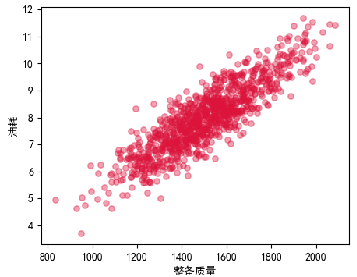（3）加油行为分布：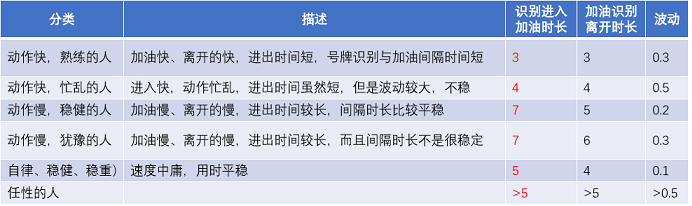（4）非油购物占比为30%，随机购物为5%，卡充值频次为10%；
（5）加油枪流速37±1L/min的流速；
（6）其他随机处理，各项数据一般设定随机0.8到1.2，以及号牌不识别等随机事件。

# 2. 数据集

## 2.2. 组合数据

C = A ∪ B C=A \cup B

c k = a i ∪ b j c_k = a_i \cup b_j

a i a_i 加油时间大于 b j b_j 的进站时间，小于 b j b_j 的出站时间。

# 3. 特征工程与数据预处理

## 3.1. 特征提取

### 3.1.1. 时间特征提取

（1）拆分时间数据为年、月、日、时、分、秒；
（2）按加油卡分组，加油时间排序，提取加油卡加油间隔时间，同理提取车辆进站间隔时间；

（3）每种组合条件下，进站与加油间隔时间（带小数的时）、加油与出站间隔时间（带小数的时）。

### 3.1.3. 周期时间性质特征提取

（1）均值
（2）标准差
（3）偏度
（4）峰度
（5）最大值、最小值

class ExploreDataAnalysis(object):
def __init__(self,datas):
# 略
def  Features_extra(self,drop_cols_name=[],times=1):
df = self.df.drop(drop_cols_name,axis=1)
# 取在同日、同一加油站相遇的次数
# groupby中的as_index=False，对于聚合输出，返回以组标签作为索引的对象。仅与DataFrame输入相关。as_index = False实际上是“SQL风格”的分组输出。
df_feature = df_feature.rename(columns={'fuel_interva':'times'})
# 取相遇次数>times的数据集
df_feature = df_feature[df_feature['times']>times].reset_index(drop=True)
# 依据相遇次数，筛选数据集
# 取均值
# 取标准差

#修改为标准差列名
df_feature1 = df_feature1.rename(columns={'fuel_time':'fuel_time_std','IC_time':'IC_time_std','Entry_time':'Entry_time_std','Dep_time':'Dep_time_std','Entry_interva':'Entry_interva_std', 'Dep_interva':'Dep_interva_std', 'Plate_interva':'Plate_interva_std','Shop_time':'Shop_time_std','Entry_pre':'Entry_pre_std','Dep_pre':'Dep_pre_std','Shop_interva':'Shop_interva_std'})
self.data_feature = df_feature
self.df_feature = df_feature

return df_feature


## 3.2. 特征分析

### 3.2.1. 特征分析对象

• 组合出现的频次：times
• 进出站时段内出现加油卡次数：combos
• 加油时长：fuel_time、fuel_time_std、…
• 进站时间的均值、标准差、最大值、最小值、偏度、峰度：Entry_time、Entry_time_std、…
• 加油时间的均值、标准差、最大值、最小值、偏度、峰度：IC_time、IC_time_std、…
• 购物时间的均值、标准差、最大值、最小值、偏度、峰度：Shop_time、Shop_time_std、…
• 出站时间的均值、标准差、最大值、最小值、偏度、峰度：Dep_time、Dep_time_std、…
• 进站与加油时间间隔的均值、标准差、最大值、最小值、偏度、峰度：Entry_interva、Entry_interva_std、…
• 加油与购物时间间隔的均值、标准差、最大值、最小值、偏度、峰度：Shop_interva、Shop_interva_std、…
• 购物与出站时间间隔的均值、标准差、最大值、最小值、偏度、峰度：Dep_interva、Dep_interva_std、…
• 进出站时间间隔的均值、标准差、最大值、最小值、偏度、峰度：Plate_interva、Plate_interva_std、…
• 进站与加油时间间隔/进出站时间间隔的均值、标准差、最大值、最小值、偏度、峰度：Entry_pre、Entry_pre_std、…
• 出站与加油时间间隔/进出站时间间隔的均值、标准差、最大值、最小值、偏度、峰度：Dep_pre、Dep_pre_std、…

### 3.2.2. 相关分析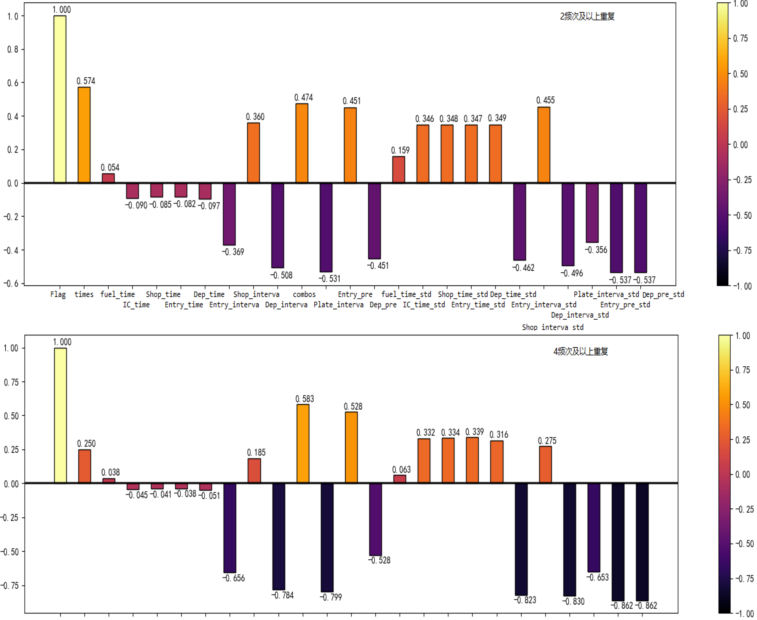### 3.2.3. 特征重要性分析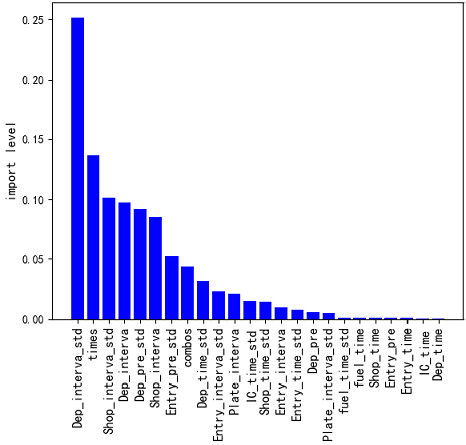def important_feature(self,cols_name):
# 模型输出目标为Flag
y_df = df[['Flag']]
X_df = df.drop('Flag',axis=1)
x_train,x_text, y_train, y_test = train_test_split(X_df,y_df,test_size=0.1)

forest_model = RandomForestClassifier(n_estimators=10, random_state=0, n_jobs=-1)
forest_model.fit(x_train, y_train)
feat_labels = X_df.columns
# feature_importances_  可以调取关于特征重要程度
importances = forest_model.feature_importances_
print("重要性：", importances)
x_columns = X_df.columns
indices = np.argsort(importances)[::-1]
x_columns_indices = []
for f in range(x_train.shape):
print("%2d) %-*s %f" % (f + 1, 30, feat_labels[indices[f]], importances[indices[f]]))
x_columns_indices.append(feat_labels[indices[f]])

print(x_columns_indices)

plt.rcParams['font.sans-serif'] = ["SimHei"]
plt.rcParams['axes.unicode_minus'] = False
plt.title("IC卡加油与号牌识别集中各个特征的重要程度", fontsize=16)
plt.ylabel("import level", fontsize=12, rotation=90)

num = len(x_columns)
for i in range(num):
plt.bar(i, importances[indices[i]], color='blue', align='center')
plt.xticks(np.arange(num), x_columns_indices, rotation=90, fontsize=12)
plt.tight_layout()
plt.show()

return importances


## 3.3. 数据分布情况分析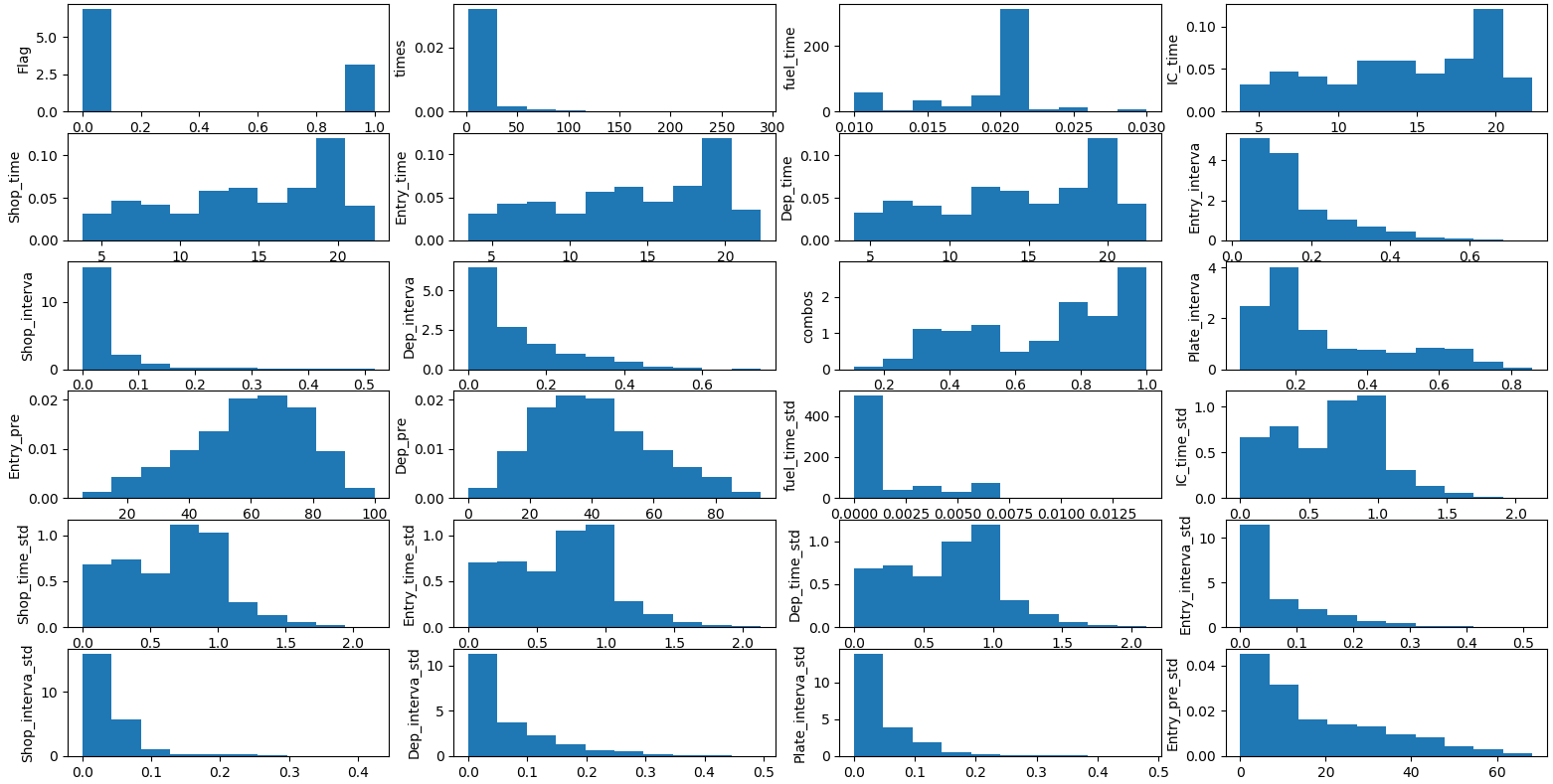def draw_Hist_KDE(self):
name = self.df_feature.columns
k= 0
fig1, ax1 = plt.subplots(nrows=6, ncols=4)
for i in range(6):
for j in range(4):
ax1[i, j].hist(self.df_feature[name[k]],density=True)

ax1[i, j].set_ylabel(name[k])
k = k + 1
plt.show()


# 4. 聚类与数据标注

    def cluster_analysis(self):
Flag=list(df['Flag'])
df=df.drop('Flag',axis=1)
samples=df.values

#标准化
scaler=StandardScaler()
kmeans=KMeans(n_clusters=2,random_state=9,precompute_distances='auto',max_iter=1000)
pipeline=make_pipeline(scaler,kmeans)
pipeline.fit(samples) #训练模型
labels=pipeline.predict(samples)#预测

df_cluster=pd.DataFrame({'labels':labels,'Flag':Flag})
ct=pd.crosstab(df_cluster['labels'],df_cluster['Flag'])
print('K-Means')
print(ct)

print('GaussianMixture')
gmm = GaussianMixture(n_components=2)
gmm.fit(samples)
labels = gmm.predict(samples)

df_cluster=pd.DataFrame({'labels':labels,'Flag':Flag})
ct=pd.crosstab(df_cluster['labels'],df_cluster['Flag'])
print(ct)

return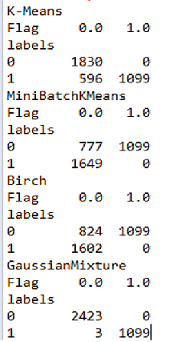# 5. 加油卡与号牌识别模型

## 5.1. 识别模型的输入与输出

### 5.1.1. 输入

（1）输入特征组成：[‘Flag’, ‘times’,‘fuel_time’,‘IC_time’,‘Entry_time’,‘Dep_time’, ‘Entry_interva’, ‘Dep_interva’,‘combos’, ‘fuel_time_std’,‘IC_time_std’,‘Entry_time_std’,‘Dep_time_std’, ‘Entry_interva_std’,‘Dep_interva_std’,‘Plate_interva’,‘Entry_pre’,‘Dep_pre’,‘Plate_interva_std’,‘Entry_pre_std’,‘Dep_pre_std’,‘Shop_time’, ‘Shop_interva’,‘Shop_interva_std’]

（2）样本数及构成，总样本数：3525，其中真值为：1099，假值为：2426，真假不平衡（未做平衡处理）

## 5.2. 集成机器学习算法XGBoostdef train_Model_fit(self,num_class,normalization=True):
params ={'learning_rate': 0.05,
'max_depth': 5,                # 构建树的深度，越大越容易过拟合
'num_boost_round':100,
'objective': 'binary:logistic', # 二分类：binary:logistic
'random_state': 7,
'silent':0,
'subsample':0.9,
'min_child_weight':5,
'eval_metric':['logloss','error','auc'], # 二分类情况
'eta':0.3
}
dtrain = xgb.DMatrix(self.x_train, label=self.y_train)
dtest = xgb.DMatrix(self.x_text,label=self.y_test)

res = xgb.cv(params,dtrain)
best_nround = res.shape - 1
watchlist = [(dtrain,'train'),(dtest,'eval')]
evals_result = {}
model = xgb.train(params,dtrain,num_boost_round=best_nround,evals = watchlist,evals_result=evals_result)
y_pred=model.predict(xgb.DMatrix(self.x_test))

model.save_model('XGboostClass.model')
predictions = [round(value) for value in y_pred]
# 计算准确率
accuracy = accuracy_score(self.y_test, predictions)
print("Accuracy: %.2f%%" % (accuracy * 100.0))

# 显示重要特征
plot_importance(model)
plt.show()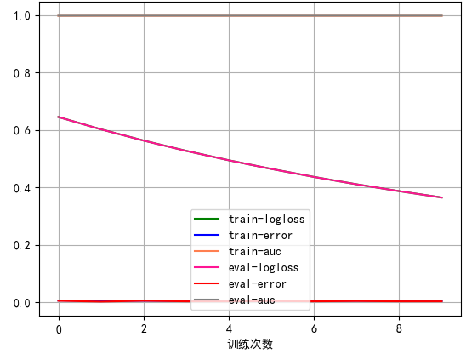## 5.2. Tensorflow BP神经网络

### 5.2.1. 神经网络结构设计

BP神经网络输入层为23个单元，接着网络各层分别为16、12、2，最后输出是2分类。关于Tensorflow神经网络各层的详解参见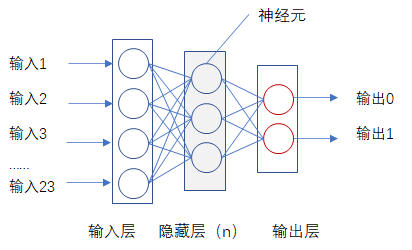• 神经元的激活函数采用relu
• 损失函数采用softmax

### 5.2.2. 网络结构代码及训练情况

（1）构建BP神经网络模型及训练代码如下

    # 输入训练数据集并设置训练与测试比例
def set_Datas(self,datas,y_cols_name,test_size=0.3):
self.datas = datas
self.y_cols_name = y_cols_name
y_df = datas[[y_cols_name]]

self.y_=pd.DataFrame(columns=('TrueIC','FalseIC'))
self.y_['TrueIC']=y_df['Flag'].apply(lambda x:0 if x==0 else 1)
self.y_['FalseIC']=y_df['Flag'].apply(lambda x:1 if x==0 else 0)
self.X_ = datas.drop(y_cols_name,axis=1)
# 归一化处理
MM = MinMaxScaler()
self.X_ = MM.fit_transform(self.X_.values )
self.y_= self.y_.values
#注意训练集、测试集返回参数顺序
self.x_train,self.x_test, self.y_train, self.y_test = train_test_split(self.X_,self.y_,test_size=test_size)

return

def train_Model_fit(self,num_class,normalization=True):
#定义神经元
def NN(h_in,h_out,layer='1'):
w = tf.Variable(tf.truncated_normal([h_in,h_out],stddev=0.1),name='weights' +layer )
b = tf.Variable(tf.zeros([h_out],dtype=tf.float32),name='biases' + layer)

return w,b

#定义BP神经网络
def BP_NN(in_units,layers=[18,10,6,2], learning_rate = 0.01):  #,dropout=True):
#定义输入变量
num = len(layers)   # 网络层数
x = tf.placeholder(dtype=tf.float32,shape=[None,in_units],name='x')
#定义输出变量
y_ = tf.placeholder(dtype=tf.float32,shape=[None,layers[num - 1]],name='y_')

#定义网络参数
w1,b1 = NN(in_units,layers,'1')   #定义第一层参数
#定义网络隐藏层
#定义前向传播过程
#定义dropout保留的节点数量
keep_prob = tf.placeholder(dtype=tf.float32,name='keep_prob')

#使用dropout
h1_drop = tf.nn.dropout(h1,rate = 1 - keep_prob)
w2,b2 = NN(layers,layers,'2')   #定义第二层参数
# 定义第二层隐藏层
w3,b3 = NN(layers,layers,'3')   #定义第三层参数
#定义损失函数
loss_func = tf.reduce_mean(-tf.reduce_sum(y_*tf.log(y_conv),reduction_indices=))
#学习率
#learning_rate = #0.01
lr = tf.Variable(learning_rate,dtype=tf.float32)

correct_pred = tf.equal(tf.argmax(y_conv,1), tf.argmax(y_,1))
accuracy = tf.reduce_mean(tf.cast(correct_pred, tf.float32))

return x,y_,loss_func,train_step,correct_pred,keep_prob,accuracy,y_conv

batch_size = 20

# 使用from_tensor_slices将数据放入队列，使用batch和repeat划分数据批次，且让数据序列无限延续
dataset = tf.data.Dataset.from_tensor_slices((self.x_train, self.y_train))
dataset = dataset.batch(batch_size).repeat()
iterator = dataset.make_one_shot_iterator()
next_iterator = iterator.get_next()

#定义神经网络的参数
in_units = 23  #输入23个特征，返回一个正确与错误
x,y_,loss_,train_step,correct_pred,keep_prob,accuracy,y_conv = BP_NN(in_units,layers=[16,12,2], learning_rate=0.01)
log_loss= []
log_acc = []
#随机梯度下降算法训练参数
with tf.Session() as sess:
sess.run(tf.global_variables_initializer())
for i in range(20000):
batch_x,batch_y = sess.run(next_iterator)
_,loss,acc = sess.run([train_step,loss_,accuracy], feed_dict={x:batch_x,y_:batch_y,keep_prob:0.8})
if i%100 == 0:
print ("step： {}, total_loss： {},accuracy： {}".format(i, loss,acc))           # 用于趋势回归，预测值
log_loss.append(loss)
log_acc.append(acc)
# 训练集验证
train_ret = sess.run(y_conv, feed_dict={x:self.x_train,keep_prob:1.0})
train_y = sess.run(tf.argmax(train_ret,1))  # 用于分类问题，取最大概率，返回训练验证结果
y_train = sess.run(tf.argmax(self.y_train,1))  # 用于分类问题，取最大概率，还原实际数据集（oneHot编码还原）
train_correct = sess.run(tf.equal(train_y,y_train)) #测试集结果对比（真/假）
# 测试集验证
ret = sess.run(y_conv, feed_dict={x:self.x_test,keep_prob:1.0})
y = sess.run(tf.argmax(ret,1))  # 用于分类问题，取最大概率，返回测试集验证结果
y_test = sess.run(tf.argmax(self.y_test,1))  # 用于分类问题，取最大概率 ，还原实际数据集（oneHot编码还原）
test_correct = sess.run(tf.equal(y,y_test)) #测试集结果对比（真/假）

print('测试集准确率：{}'.format(sess.run(tf.reduce_mean(tf.cast(test_correct, tf.float32)))))
print('训练集准确率：{}'.format(sess.run(tf.reduce_mean(tf.cast(train_correct, tf.float32)))))

return


（2）训练参数与过程

BP神经网络模型，训练过程如下图所示，其中，训练20000次，每次batch=20，学习率为0.01，而且使用Sklearn的MinMaxScaler对数据做归一化处理。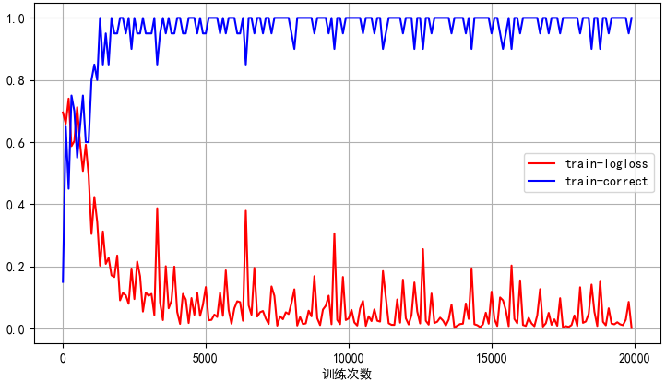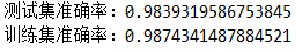# 6. 总结

《大数据人工智能常用特征工程与数据预处理Python实践（2）》 CSDN博客 ，肖永威 ，2020年12月
.《Tensorflow BP神经网络多输出模型在生产管理中应用实践》 CSDN博客 ， 肖永威 ，2020年9月
.《XGBoost线性回归工控数据分析实践案例（原生篇）》 CSDB博客 ， 肖永威 ，2020年8月
.《浅谈项目管理结构化思维》 CSDN博客 ， 肖永威 ，2020年4月

09-104390
02-13486801-061万+
10-044367
12-065125
05-305920
10-233万+
09-121万+
01-04150
07-254312
10-291240
03-0630
05-22612
10-252万+
02-15
09-28
01-051万+